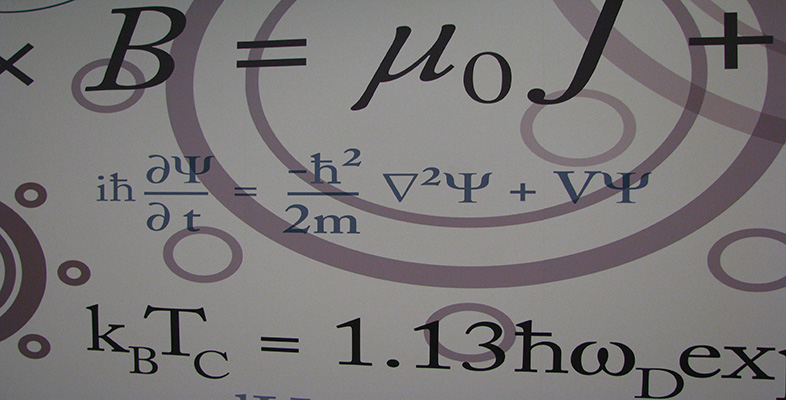Number systems

This free course is available to start right now. Review the full course description and key learning outcomes and create an account and enrol if you want a free statement of participation.

Free course

1 Real numbers

1.1 Rational numbers

In OpenLearn course M208_5 Mathematical language you met the sets

•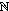= {1, 2, 3, …}, the natural numbers;

•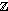= {…, −2, −1, 0, 1, 2, …}, the integers;

•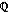= {p/q : p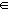, q}, the rational numbers.is the set of numbers that can be written as fractions, such asand –1.7 =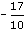.

Notice that each set in this list is a subset of the succeeding one; that is,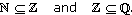To represent the rational numbers geometrically, we use a number line. We begin by drawing a line and marking on it points corresponding to the integers 0 and 1. If the distance between 0 and 1 is taken as a unit of length, then the rational numbers can be arranged on the line in a natural order. For example, the rational 4/3 is placed at the point which is one third of the distance from 1 to 2.

We sometimes use ‘rational’ as shorthand for ‘rational number’ and ‘real’ for ‘real number’.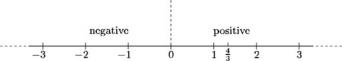Each rational number also has a decimal representation, which is either a terminating (that is, finite) decimal, such as 1.54, or a recurring decimal such as 2.134 734 734 7 …, in which the digits repeat in a regular pattern from some position onwards. The decimal representation of any rational number p/q can be obtained by using long division to divide q into p.

One of the surprising mathematical discoveries made by the ancient Greeks was that the system of rational numbers is not adequate to describe all the lengths that occur in geometry. For example, consider the diagonal of a square of side 1. What is its length? If the length is x, then, by Pythagoras' Theorem, x must satisfy the equation x2 = 2. However, there is no rational number that satisfies this equation.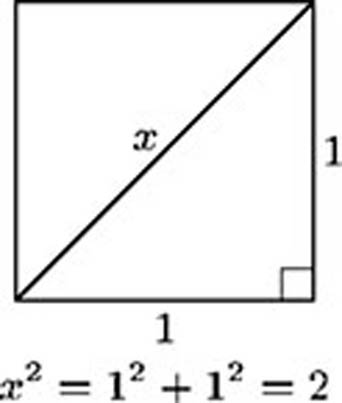Theorem 1

There is no rational number x such that x2 = 2.

Proof   Suppose that such a rational number x exists. Then we can write x = p/q, where p, q. By cancelling, if necessary, we may assume that the greatest common factor of p and q is 1.

This is a proof by contradiction.

The equation x2 = 2 now becomes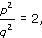so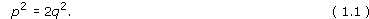The square of an odd number is odd, so p cannot be odd. Hence p is even, so we can write p = 2r, say. Equation (1.1) now becomes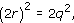so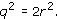We have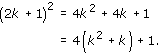Reasoning as before, we find that q is also even.

Since p and q are both even, they have a common factor of 2, which contradicts our earlier statement that the greatest common factor of p and q is 1.

Since we have obtained a contradiction, our original assumption must be false; therefore no such rational number x exists.

Exercise 1

By imitating the proof above, show that there is no rational number x such that x3 = 2.

Solution

Suppose that there exists a rational number x such that x3 = 2. We can write x = p/q, where p and q are positive integers whose greatest common factor is 1. Then the equation x3 = 2 becomes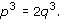Now the cube of an odd number is odd because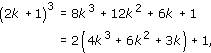so p must be even. If we write p = 2r, then our equation becomes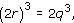so we have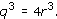Hence q is also even, so 2 is a common factor of p and q. This contradiction shows that such a number x cannot exist.

Since we expect equations such as x2 = 2 and x3 = 2 to have solutions, we must introduce new numbers that are not rationals. We denote the positive solutions of these two equations by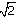, and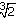, respectively; thus ()2 = 2 and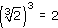. Of course, we must introduce many other new numbers, such as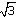,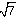, and so on. Indeed, it can be shown that if m and n are natural numbers, and the equation xm = n has no integer solution, then the positive solution of this equation, written as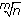, cannot be rational.

The case m = 2 and n = 3 is treated in Exercise 5.

There are many other mathematical quantities which cannot be described exactly by rational numbers. For example, the number, which denotes the area of a disc of radius 1 (or half the length of the perimeter of such a disc), cannot be described by a rational number, and neither can the number e.

Johann Heinrich Lambert proved in 1761 thatis irrational. He was a colleague of Euler and Lagrange at the Berlin Academy of Sciences, and is best known for his work on.

All these numbers are known as irrational numbers.

M208_6